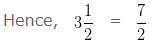# Types of Fraction | Proper | Improper | Mixed Fraction

Types of Fraction:

• Proper Fraction
• Improper Fraction
• Mixed Fraction
– Convert Improper Fraction To Mixed Fraction
– Convert Mixed Fraction To Improper Fraction

## Fraction:

• A fraction is a part of the whole number.
• In generalising form for any two natural numbers ‘a’ and ‘b’ fraction is:
• a/b

### Where,

• a = Numerator
• b = Denominator

### Example 1

Write down the Numerator and Denominator of the Fraction:

4/3

### Explanation

The numerator is the upper part (Number on Top) of the Fraction

So, the Numerator of 4/3 is 4

The denominator is the lower number ( Number at Bottom) of the Fraction
So, the Denominator of 4/3 is 3

### Example 2

Write down the Numerator and Denominator of the Fraction:

5/6

### Explanation

The numerator is the upper part (Number on Top) of the Fraction
So, the Numerator of 5/6 is 5

The denominator is the lower number ( Number at Bottom) of the Fraction
So, the Denominator of 5/6 is 6

### TYPES OF FRACTION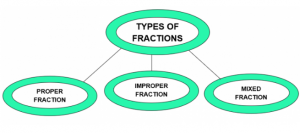### Types of Fraction – Proper Fraction

• A proper fraction is a fraction which represents the part of the whole number. In a proper fraction, the numerator is always less than its denominator.
• In generalising form for any two integers ‘a’ and ‘b’
• a/b where (a < b)
• i.e Numerator < Denominator

### Example 1

Is 2/3 is a proper fraction?

### Explanation

A proper fraction is a fraction which represents the part of the whole number. In a proper fraction, the numerator is always less than its denominator.

• In generalise form for any two integer ‘a’ and ‘b’
• a/b where (a < b)
• i.e Numerator < Denominator
• Numerator = 2
• Denominator = 3
• Here 2 < 3
• i.e, Numerator < Denominator
• Hence, 2/3 is a proper fraction

### Example 2

Is 7/5 is a proper fraction?

### Explanation

A proper fraction is a fraction which represents the part of the whole number. In a proper fraction, the numerator is always less than its denominator.

• In generalising form for any two integers ‘a’ and ‘b’
• a/b where (a < b)
• i.e Numerator < Denominator
• Numerator = 7
• Denominator = 5
• Here 7 > 5
• i.e, Numerator > Denominator
• Hence, 7/5 is not a proper fraction

### Types of Fraction – Improper Fraction

Improper fractions are the combination of a whole number and a proper fraction. An improper fraction is opposite of proper fraction since in improper fraction numerator is greater than its denominator

• In generalising form for any two integers ‘a’ and ‘b’
• a/b where (a > b)
• i.e Numerator > Denominator

### Example 1

Is 17/9 is an improper fraction?

### Explanation

Improper fractions are the combination of a whole number and a proper fraction. An improper fraction is opposite of proper fraction since in improper fraction numerator is greater than its denominator

• In generalising form for any two integers ‘a’ and ‘b’
• a/b where (a > b)
• i.e Numerator > Denominator
• Numerator = 17
• Denominator = 9
• Here, 17 > 9
• i.e, Numerator > Denominator
• Hence, 17/9 is an improper fraction.

### Example 2

Is 7/10 is an improper fraction?

### Explanation

Improper fractions are the combination of a whole number and a proper fraction. An improper fraction is opposite of proper fraction since in improper fraction numerator is greater than its denominator

• In generalising form for any two integers ‘a’ and ‘b’
• a/b where (a > b)
• i.e Numerator > Denominator
• Numerator = 7
• Denominator = 10
• Here, 7 < 10
• i.e, Numerator < Denominator
• Hence, 7/10 is not an improper fraction.

### Types of Fraction – Mixed Fraction

Mixed Fraction is a sum of a whole number and a proper fraction. An improper fraction can be converted into a mixed fraction and mixed fraction can be converted into an improper fraction with the help of simple multiplication, division and addition.

### Convert Improper Fraction to Mixed Fraction

To Convert Improper Fraction into Mixed Fraction, we follow the steps given below:-

Step I: Divide the Numerator by the Denominator.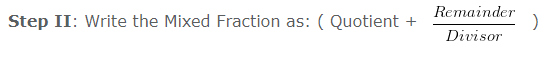### Example 1

Convert 3/2 into Mixed Fraction.

### Explanation

Given Fraction:

• 3/2
• Divide the Numerator by the Denominator
• On dividing 3 by 2
• We get Quotient = 1
• Remainder = 1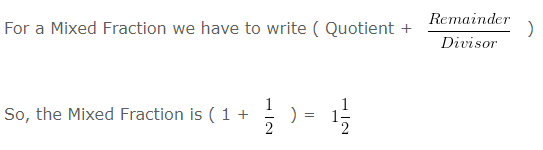### Example 2

Convert 5/3 into Mixed Fraction.

### Explanation

Given Fraction:

• 5/3
• Divide the Numerator by the Denominator
• On dividing 5 by 3
• We get Quotient = 1
• Remainder = 2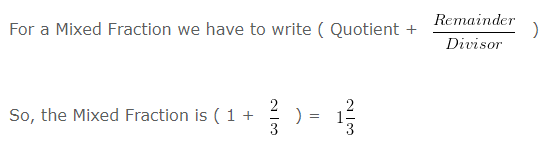### Convert Mixed Fraction to Improper Fraction

To Convert Mixed Fraction into Improper Fraction, we follow the steps given below:-

Step 1: Multiply the Whole Number Part and Denominator of the Mixed Fraction

Step 2: Add the Numerator to the product obtained in Step 1

Step 3: Write the sum as the numerator and denominator would remain the same, as in the Mixed Fraction.

### Example 1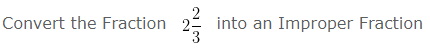### Explanation

A combination of Whole Number and a Proper Fraction is called a Mixed Fraction Here,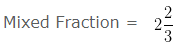• Whole Number Part = 2
• Numerator = 2
• Denominator = 3
• Step 1: Multiply the Whole Number Part and Denominator of the Mixed Fraction
• 2 x 3 = 6
• Step 2: Add the Numerator to the product obtained in Step 1
• 2 + 6 = 8
• Write the sum as the numerator and denominator would remain the same, as in the Mixed Fraction.
8/3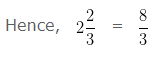### Example 2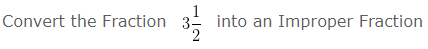### Explanation

A combination of Whole Number and a Proper Fraction is called a Mixed Fraction Here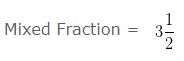• Whole Number Part = 3
• Numerator = 1
• Denominator = 2
• Step 1: Multiply the Whole Number Part and Denominator of the Mixed Fraction
• 3 x 2 = 6
• Step 2: Add the Numerator to the product obtained in Step 1
• 1 + 6 = 7
• Write the sum as the numerator and denominator would remain the same, as in the Mixed Fraction.
• 7/2